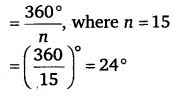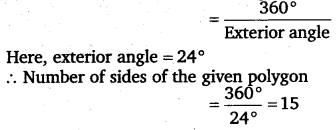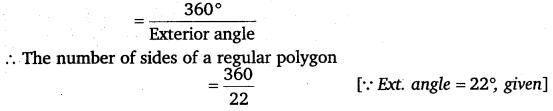# Class 8 Maths NCERT Solutions for Chapter – 3 Understanding Quadrilaterals Ex – 3.2

Question 1.
Find x in the following figures.Solution.
We know that the sum of the exterior angles formed by producing the sides of a convex polygon in the same order is equal to 360°. Therefore,
(a) x + 125° + 125° = 360°
⇒ x + 250° = 360°
⇒ x = 360° – 250° = 110°
(b) x + 90° +60° + 90° + 70° = 360°⇒  x + 310° = 360°
⇒  x = 360° – 310° = 50°

Question 2.
Find the measure of each exterior angle of a regular polygon of
(i)
9 sides
(ii) 15 sides

Solution.
(i) Each exterior angle of a regular polygon of 9 sides(ii) Each exterior angle of a regular polygon of 15 sidesQuestion 3.
How many sides does a regular polygon have if the measure of an exterior angle is 24°?

Solution.
Since the number of sides of a regular polygonQuestion 4.
How many sides does a regular polygon have if each of its interior angles is 165°?

Solution.
Let there be n sides of the polygon. Then, its each interior angleThus, there are 24 sides of the polygon.

Question 5.
(a) Is it possible to have a regular polygon with measure of each exterior angle as 22°?
(b) Can it be an interior angle of a regular polygon ? Why?

Solution.
(a) Since the number of sides of a regular polygon$\frac { 180 }{ 11 }$,
Which is not a whole number.
A regular polygon with measure of each exterior angle as 22° is not possible.

(b) If interior angle = 22°, then its exterior angle = 180° – 22° = 158°.
But 158 does not divide 360 exactly.
Hence, the polygon is not possible.

Question 6.
(a) What is the minimum interior angle possible for a regular polygon? Why?
(b) What is the maximum exterior angle possible for a regular polygon?

Solution.
(a) The equilateral triangle being a regular polygon of 3 sides has the least measure of an interior angle = 60°.
(b) Since the minimum interior angle of a regular polygon is equal to 60°, therefore, the maximum exterior angle possible for a regular polygon = 180° – 60° – 120°.

#### Class 8 Maths NCERT Solutions for Chapter – 3 Understanding Quadrilaterals Ex – 3.3

Lorem ipsum dolor sit amet, consectetur adipiscing elit. Phasellus cursus rutrum est nec suscipit. Ut et ultrices nisi. Vivamus id nisl ligula. Nulla sed iaculis ipsum.

Company Name